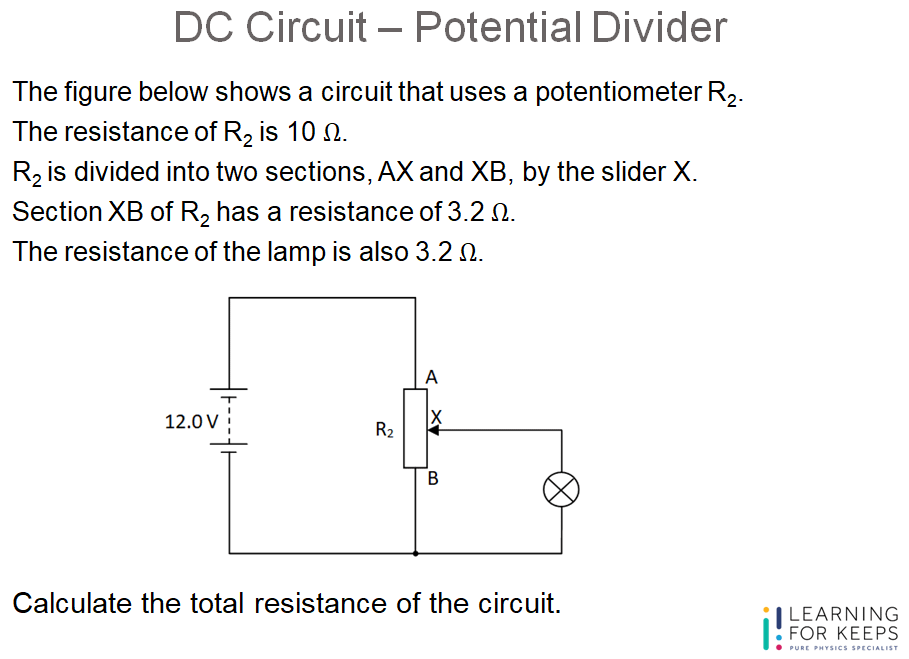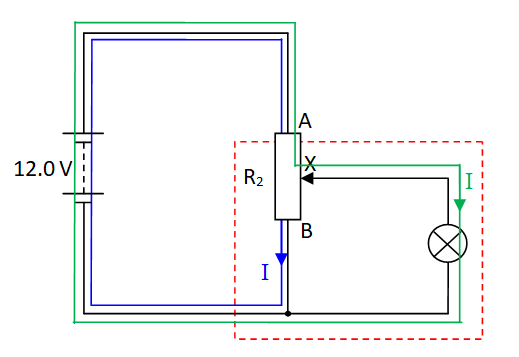# Question Guide – DC Circuit – Potential Divider

Question:
The figure below shows a circuit that uses a potentiometer R2. The resistance of R2 is 10 Ω. R2 is divided into two sections, AX and XB, by the slider X. Section XB of R2 has a resistance of 3.2 Ω. The resistance of the lamp is also 3.2 Ω. Calculate the total resistance of the circuit.Solutions are open to final year Learning For Keeps students who are preparing for the upcoming O Level exam only. Please log in to see the authorized content in this sections:

Observation:
Firstly, identify whether it is a series or parallel circuit overall. Overall, it is a series circuit where the lower section contains a parallel sub-circuit.Solution:This question falls under the topic DC Circuit – Potential Divider in Physics tuition for O Level.

DC Circuit Potential Divider Sample Question and Solution ultima modifica: 2017-10-13T08:49:56+00:00 da